# 算法设计的要求

#### 正确性

1. 算法程序没有语法错误；

2. 算法程序对于合法的输入数据能够产生满足要求的输出结果；

3. 算法程序对于非法的输入数据能够得出满足规格说明的结果；

4. 算法程序对于精心选择的，甚至是刁难的测试数据都有满足要求的输出结果；

# 算法时间复杂度

## 前言

#### 1. 忽略加法常数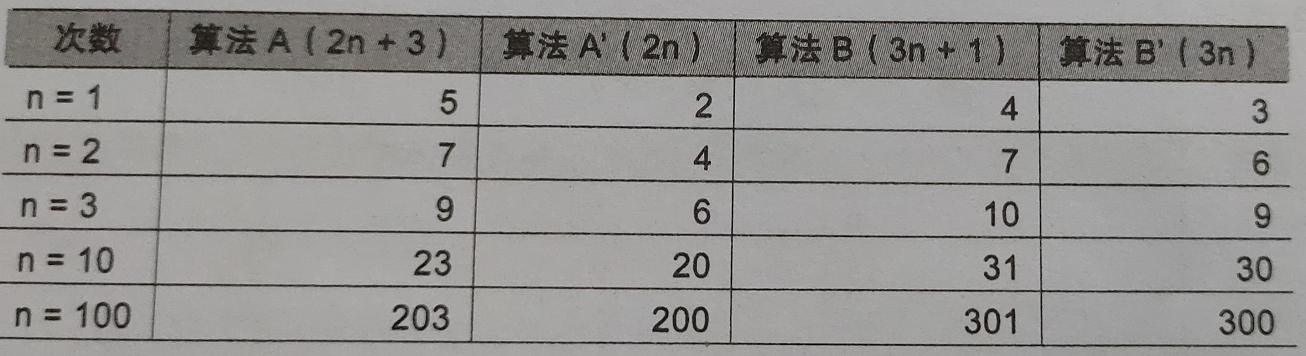#### 2. 与最高次项相乘的常数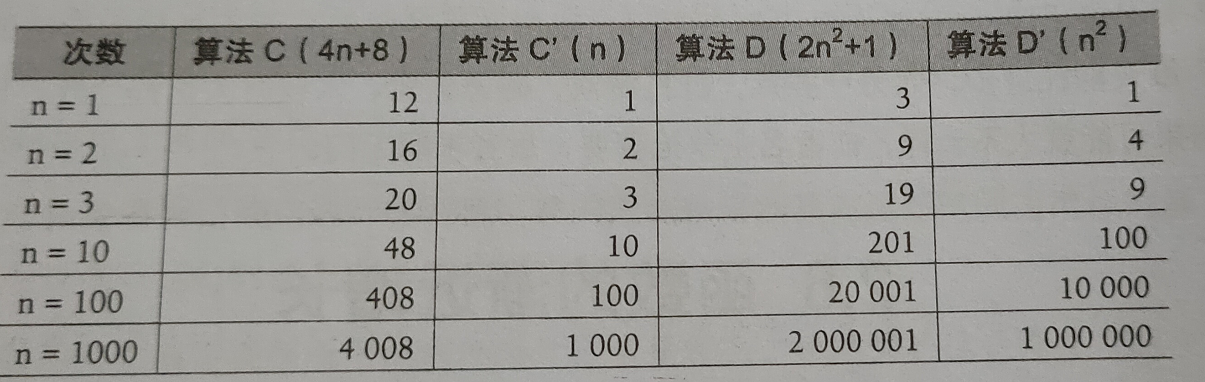#### 3. 最高次项的指数越大，增长速度越快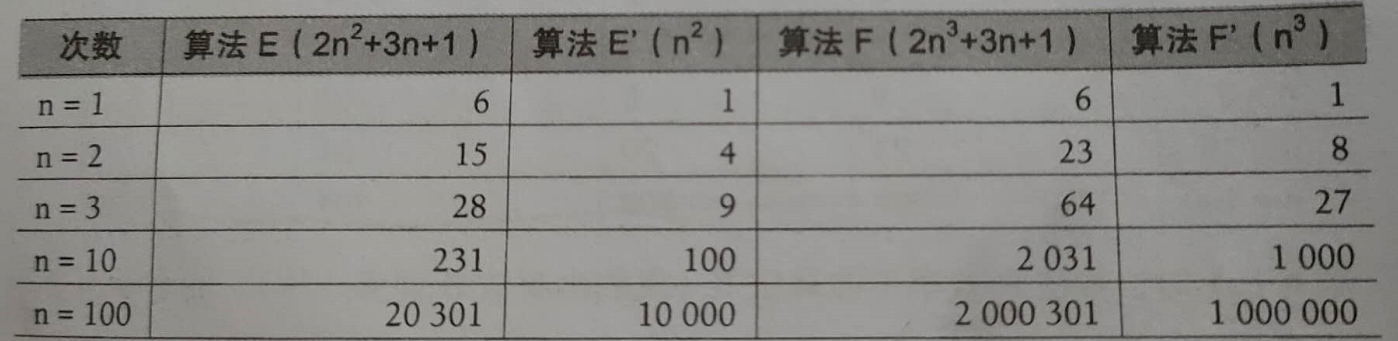#### 4. 关注主项（最高阶项）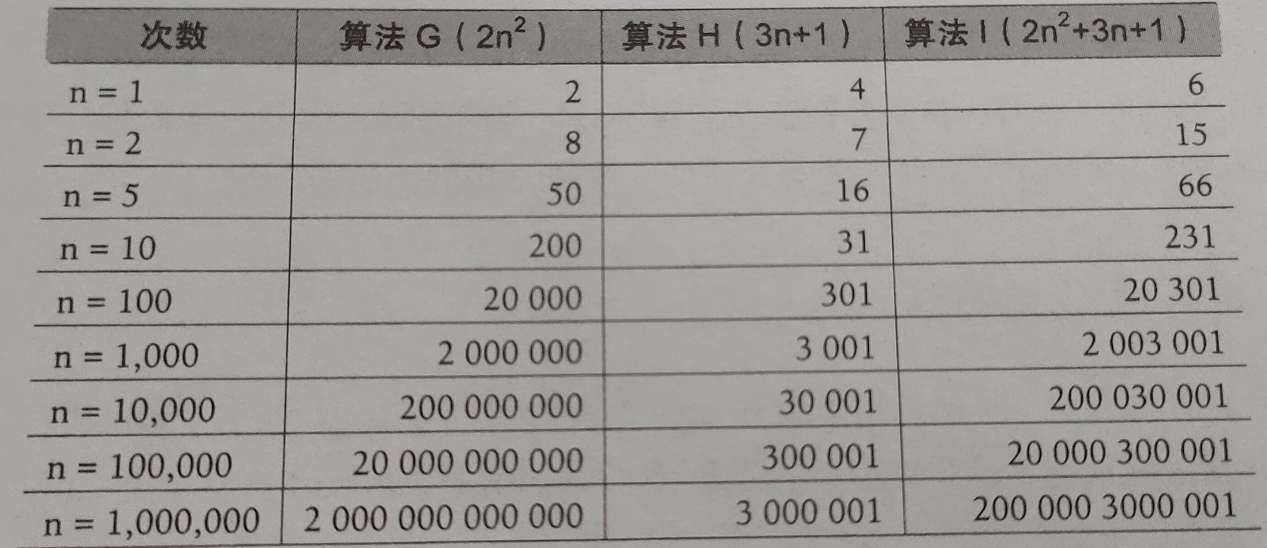## 推导大O阶方法

• 用常数1取代运行时间中所有的加法常数。

• 在修改后的运行次数函数中，只保留最高阶项。

• 如果最高阶存在且不为1，则去除与这个项相乘的常数。

#### 常数阶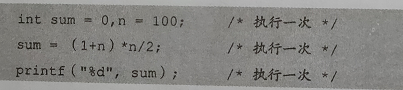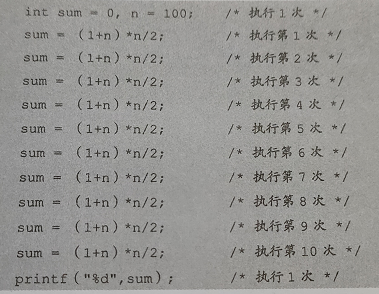#### 线性阶

int i;
for(i = 0; i < n; i++)
{
/* 时间复杂度为o(1)的程序步骤序列 */
}


#### 对数阶

int count = 1;
for(i = 0; i < n; i++)
{
count = count * 2;
/* 时间复杂度为o(1)的程序步骤序列 */
}


#### 平方阶

int i,j;
for(i = 0; i < n; i++)
{
for(j = 0; i < n; j++)
{
/* 时间复杂度为o(1)的程序步骤序列 */
}
}


int i,j;
for(i = 0; i < n; i++)
{
// 注意这里j=i，而不是0
for(j = i; j < n; j++)
{
/* 时间复杂度为o(1)的程序步骤序列 */
}
}


n+(n-1)+(n-2)+...+1 = n(n+1)/2 = (n^2)/2 + n/2©️2019 CSDN 皮肤主题: 编程工作室 设计师: CSDN官方博客